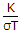# Electronics and Communication Engineering - Materials and Components

### Exercise :: Materials and Components - Section 4

6.

The dielectrics having only one kind of atoms are known as elemental dielectrics.

 A. True B. False

Explanation:

No answer description available for this question. Let us discuss.

7.

For a core having a relative permeability μr, magnetic dipole moment per unit volume M and field strength H, the flux density B is

 A. B = μr (H + M) B. B = μ0 (H + M) C. B = μ0μr (H + M) D. B = μ0μr (H - M)

Explanation:

No answer description available for this question. Let us discuss.

8.

The law that(where K is thermal conductivity, σ is electrical conductivity and T is absolute temperature) for all metals is equal to a universal constant is known as

 A. Lorentz law B. Wiedemann Franz law C. Curie Weiss law D. Kittel law

Explanation:

No answer description available for this question. Let us discuss.

9.

In elemental dielectrics, the orientation and ionic polarization are absent.

 A. True B. False

Explanation:

No answer description available for this question. Let us discuss.

10.

In which of the following semiconductor are holes majority carriers

 A. intrinsic B. extrinsic C. p type D. n type